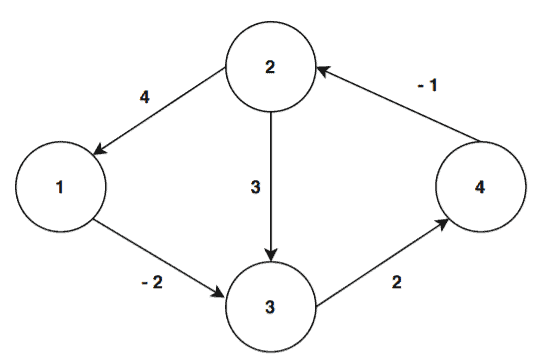## 1. Overview

In this tutorial, we’ll discuss the Floyd-Warshall Algorithm, and then we’ll analyze its time complexity.

## 2. Floyd-Warshall Algorithm

The Floyd-Warshall algorithm is a popular algorithm for finding the shortest path for each vertex pair in a weighted directed graph.

In all pair shortest path problem, we need to find out all the shortest paths from each vertex to all other vertices in the graph.

Now, let’s jump into the algorithm:We’re taking a directed weighted graphas an input. And first, we construct a graph matrix from the given graph. This matrix includes the edge weights in the graph.

Next, we insertin the diagonal positions in the matrix. The rest of the positions are filled with the respective edge weights from the input graph.

Then, we need to find the distance between two vertices. While finding the distance, we also check if there’s any intermediate vertex between two picked vertices. If there exists an intermediate vertex then we check the distance between the selected pair of vertices which goes through this intermediate vertex.

If this distance when traversing through the intermediate vertex is less then the distance between two picked vertices without going through the intermediate vertex, we update the shortest distance value in the matrix.

The number of iterations is equal to the cardinality of the vertex set. The algorithm returns the shortest distance from each vertex to another in the given graph.

## 3. An Example

Let’s run the Floyd-Warshall algorithm on a weighted directed graph:At first, we construct a graph matrix from the input graph. Next, we insertto the diagonal positions in the matrix, and the rest of the positions will be filled with the edge weights from the input graph:Now, we’re ready to start the iteration. The cardinality of the vertex set is. We’ll iterate the loopstimes.

Let’s start with the first loop. For the first loop k =1, i=1, j= 1 we’ll check if we should update the matrix:>>As the loop values don’t satisfy the condition, there will be no update in the matrix.

Let’s continue, now for the values k =1, i=1, j= 2 and check again:>>Thus, there will be no changes in the matrix. In this way, we’ll continue and check all pair of vertices.

Let’s fast-forward to some values that will satisfy the distance condition.

For the loop values k =1, i=2, j= 3, we’ll see that the condition is satisfied:>>>Because of that, we’ll compute a new distance:Hence, the condition satisfies for the vertex pair. At first, the distance between the vertextowas. However, we found a new shortest distancehere. Because of that, we update the matrix with this new shortest path distance:Let’s take another set of values for the three nested loops such that the loop values satisfy the distance condition given in the algorithm; k=2, i= 4, j= 1:>>>As the condition satisfies, we’ll calculate a new distance calculation:Therefore, we update the matrix now with this new value:Similarly, we continues and checks for different loop values. Finally, after the algorithm terminates, we’ll get the output matrix containing all pair shortest distances:## 4. Time Complexity Analysis

First, we inserted the edge weights into the matrix. We do this using a for loop that visits all the vertices of the graph. This can be performed intime.

Next, we’ve got three nested loops, each of which goes from one to the total number of vertices in the graph. Hence, the total time complexity of this algorithm is.

## 5. Conclusion

To summarize, in this tutorial, we’ve discussed the Floyd-Warshall algorithm to find all pair shortest distance in a weighted directed graph.

Furthermore, we’ve also presented an example and time complexity analysis of the algorithm.